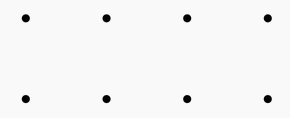###### back to index | new

Let $A={1,2,3,4}$, and $f$ and $g$ be randomly chosen (not necessarily distinct) functions from $A$ to $A$. Find the probability that the range of $f$ and the range of $g$ are disjoint.

How many non-congruent triangles have vertices at three of the eight points in the array shown below?How many ways can all six numbers in the set $\{4, 3, 2, 12, 1, 6\}$ be ordered so that $a$ comes before $b$ whenever $a$ is a divisor of $b$?

How many collections of six positive, odd integers have a sum of $18$? Note that $1 + 1 + 1 + 3 + 3 + 9$ and $9 + 1 + 3 + 1 + 3 + 1$ are considered to be the same collection.